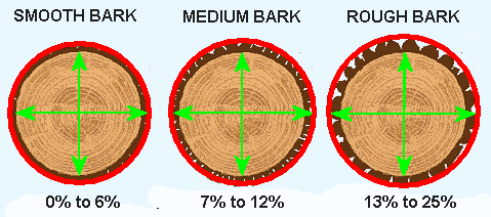Spike's Calculators

# Estimate the Volume of Bark as a Percentage CalculatorThe volume of bark on a tree varies by species and will vary along the length of the tree. It is almost impossible to calculate exact values. Average bark volume can be estimated with the Dobie and Wright's formula.

This formula will estimate the average bark volume as a percentage of the total volume and as a percentage of the wood volume.### Estimating Void Areas

Not all bark is the same. You will have to estimate the void area of the bark.Diameter measurements can be entered in units of inches, centimetres or millimetres.

Diameter Measured Outside Bark in
Diameter Measured Inside Bark in
Bark Voids %

#### Results:

 Bark as a percentage of total volume % Bark as a percentage of total volume Minus Voids % Bark as a percentage of wood volume % Bark as a percentage of wood volume Minus Voids %